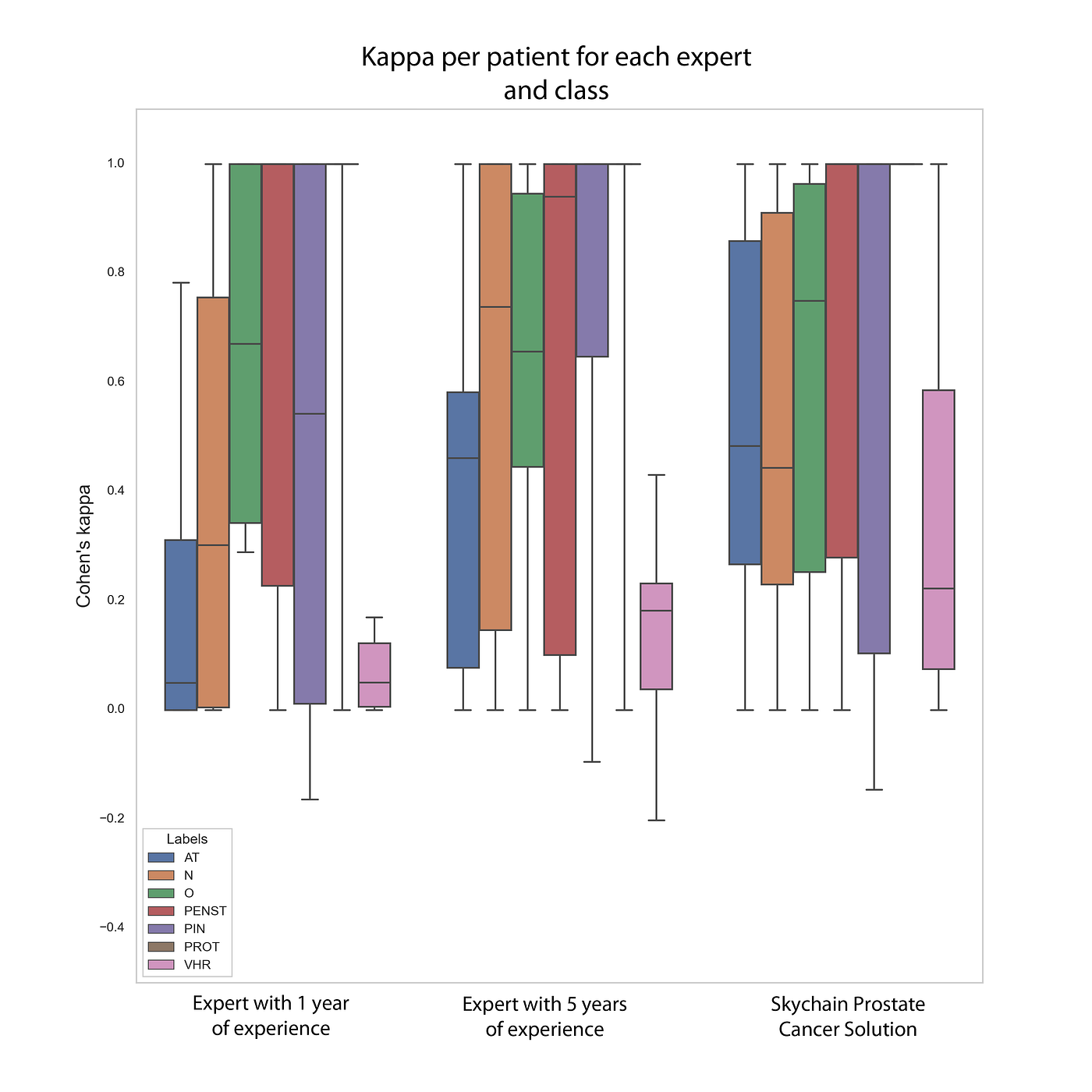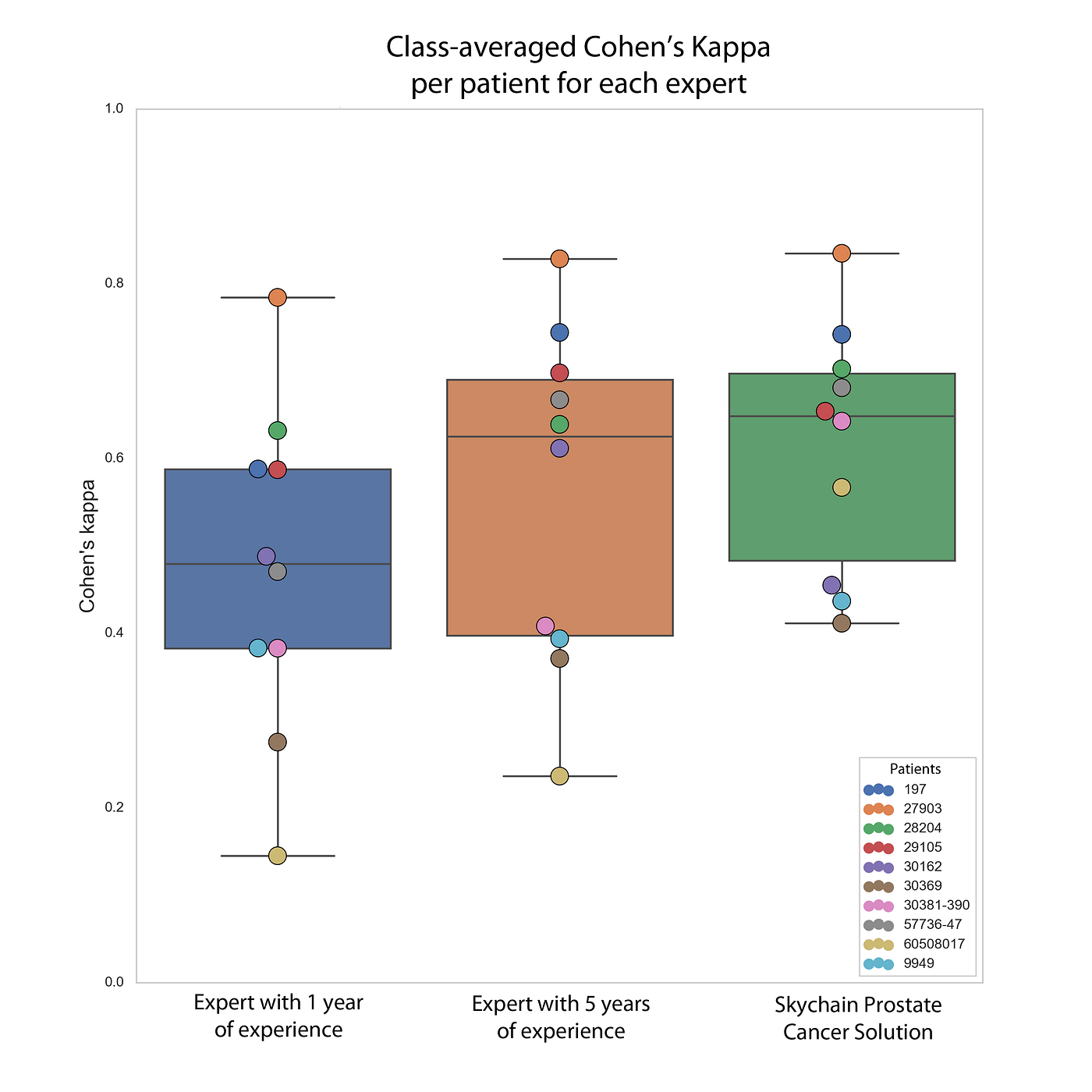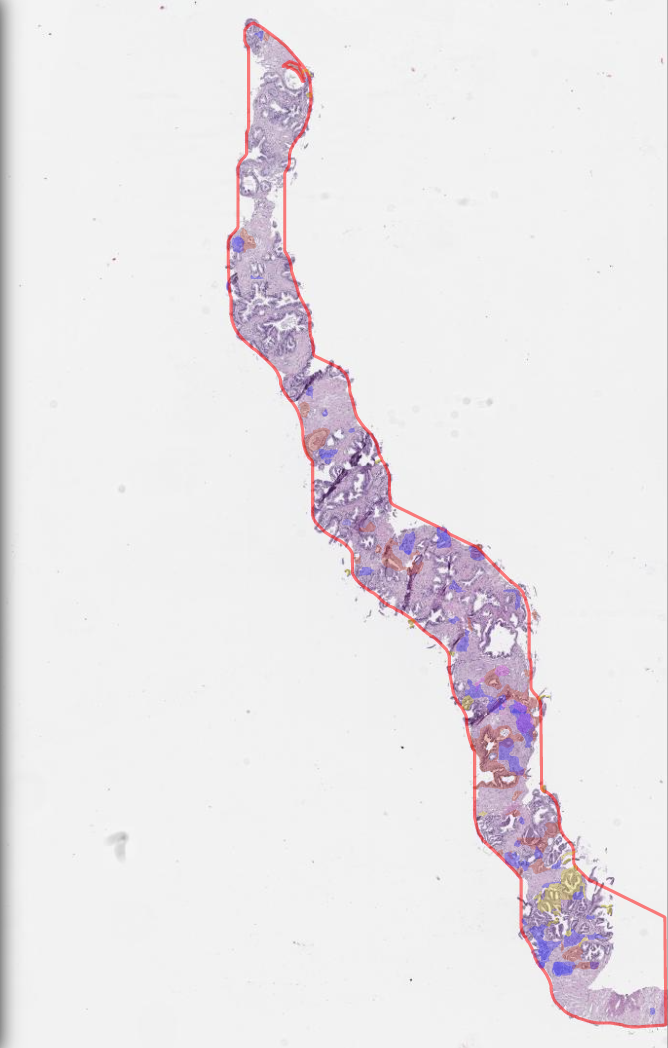# Results

This is the example of doctor’s opinion on one of the patients:
Slide 1: O, PIN, Gleason Score 3 + 4 = 7, occupies 30% of the punctate length.
Slide 2: O, PIN, Gleason Score 4 + 3 = 7, occupies 100% of the punctate length.
Slide 3: O, Gleason Score 3 + 4 = 7 points, occupies 90% of the punctate length.
Slide4: O, PIN, Gleason Score 3 + 3 = 6 points, occupies 90% of the punctate length.
Slide 5: O, PIN, Gleason Score 3 + 3 = 6 points, occupies 90% of the punctate length.
Slide 6: O, PIN, Gleason Score 3 + 3 = 6 points, occupies 80% of the punctate length.
Slide 7: O, Gleason Score 4 + 4 = 8 points, occupies 50% of the punctate length.
Slide 8: O, Gleason Score 4 + 3 = 7 points, occupies 40% of the punctate length.
Slide 9: O, PIN, Gleason Score 4 + 4 = 8 points, takes 50% of the punctate length.
Slide 10: O, Gleason Score 5 + 5 = 10 points, occupies 40% of the punctate length.
Slide 11: O, Gleason Score 5 + 5 = 10 points, occupies 90% of the punctate length.
Slide 12: N, small columns of prostate tissue without tumor growth.

Explanation: Cohen’s kappa measures agreement between two evaluators, each classifying N elements in C mutually exclusive categories. In our case, the Cohen’s kappa will be calculated on 108 test slides for N experts + the prediction of our neural network, that is, we will get N + 1 values.

Cohen’s Kappa Formula:

where pо is the relative observable agreement between the evaluators (identical in accuracy), and pе is the hypothetical probability of a random agreement, using the observed data to calculate the likelihood of each observer randomly seeing each category.X-axis — doctors making a diagnosis + neural network, Y-axis — Cohen’s kappa. This diagram shows the distribution of Cohen’s kappa for 2 doctors and the neural network. The legend on the bottom left in color indicates the belonging to each of the classes. The graph displays the minimum / maximum value. The body of the candlestick is calculated as the median value + — the standard deviation.X-axis — doctors making a diagnosis + neural network, Y-axis — Cohen’s kappa. The different colors correspond to different doctors for clarity.

# Curious caseTissue sample. As you can see, it has some few blue areas, which mean that Skychain neural network sees it as cancer.

--

--